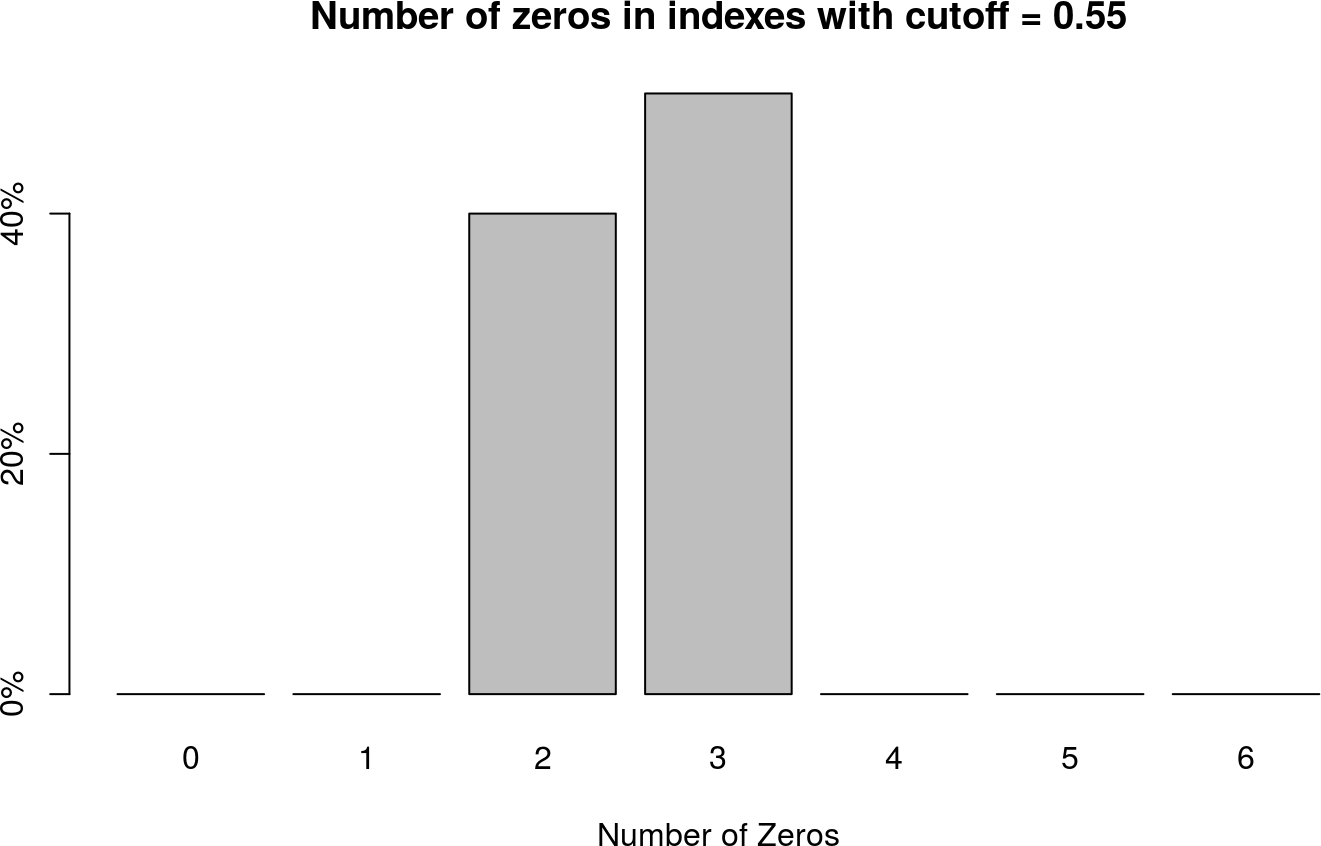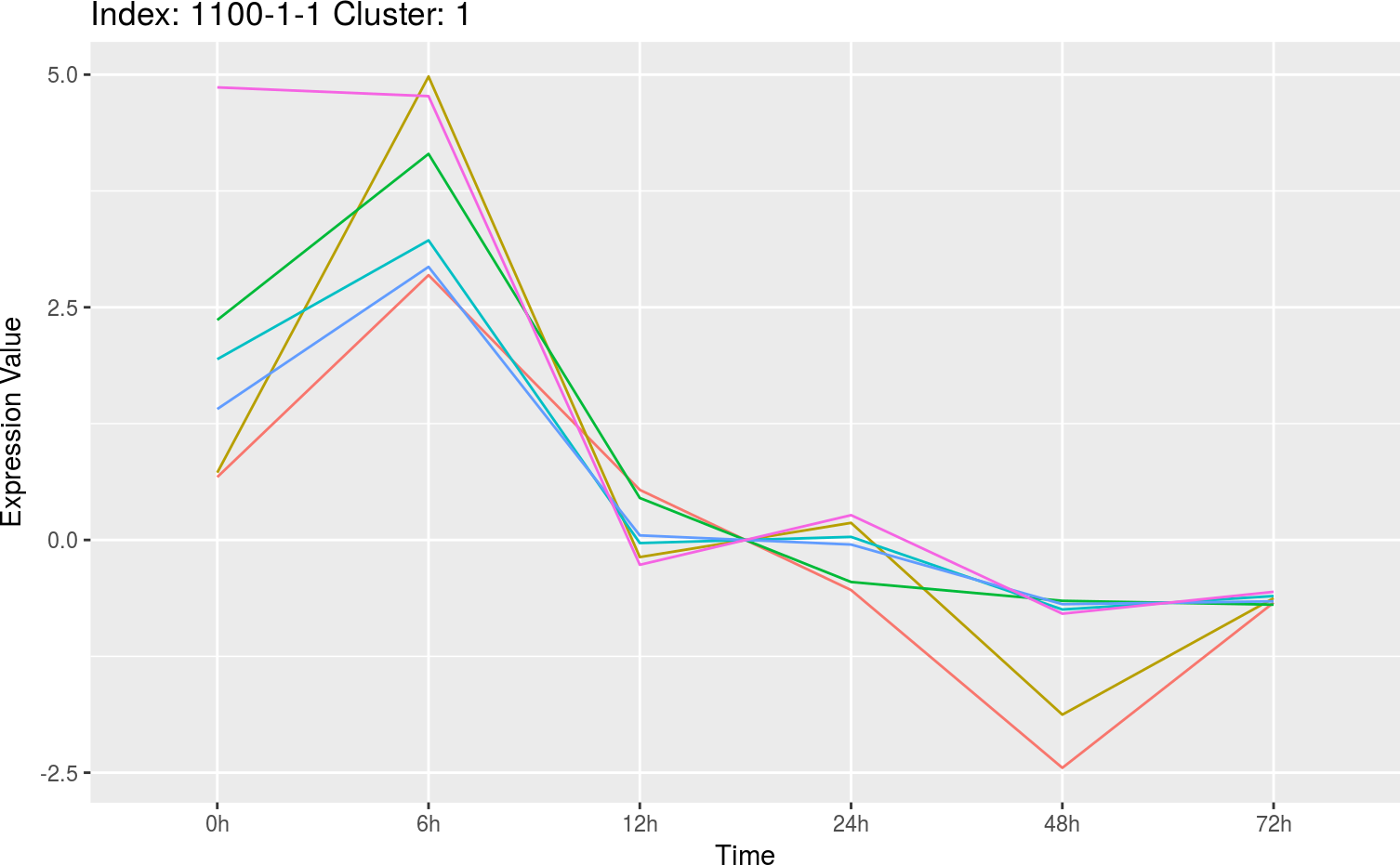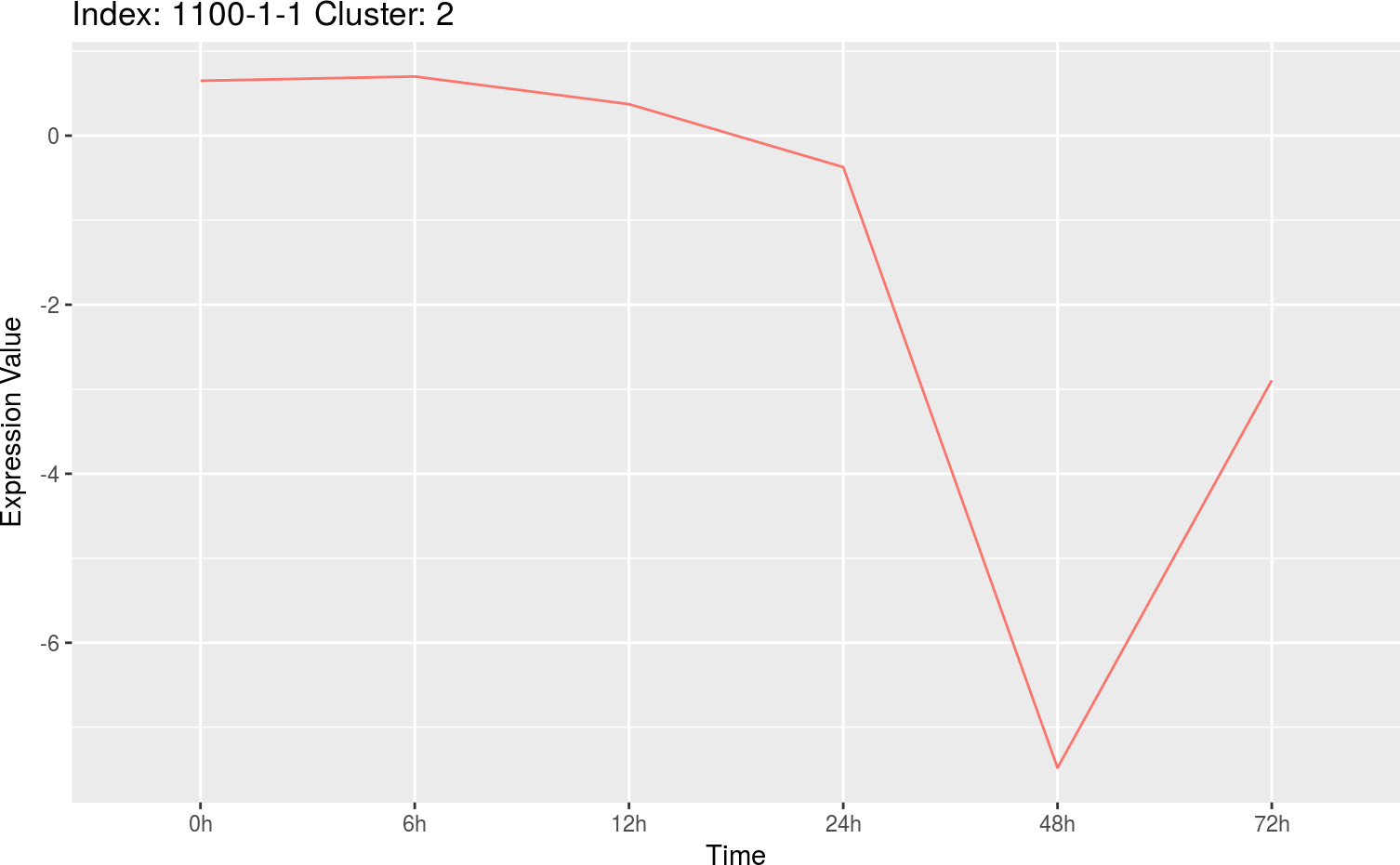# 1 Installing ctsGE

source("https://bioconductor.org/biocLite.R")
biocLite("ctsGE")

# 2 Workflow of clustering with ctsGE

1. Build the expression matrix from expression data

2. Define an expression index (i.e., a sequence of 1,-1, and 0) for each gene

3. Cluster the gene set with the same index, applying K-means: $kmeans(genesInIndex,k)$

4. Graphic visualization of expression patterns

5. Interactive visualization and exploration of gene-expression data

# 3 Building the expression matrix

As input, the ctsGE package expects a normalized expression table, where rows are genes and columns are samples. This can consist of count data as obtained, e. g., from RNA-Seq or other high-throughput sequencing experiment or microarray experiment. Example data from the [Gene Expression Omnibus (GEO)] (http://www.ncbi.nlm.nih.gov/geo/query/acc.cgi?acc=GSE2077) are used here illustrate ctsGE’s potential. The data are the expression profile of Cryptosporidium parvum-infected human ileocecal adenocarcinoma cells (HCT-8),3 Deng M, Lancto CA, Abrahamsen MS (2004). Cryptosporidium parvum regulation of human epithelial cell gene expression. Int. J. Parasitol. 34, 73–82.) comprised of 12,625 genes over 18 samples (three replicates of six developmental stages in human cancer). For tutorial purposes and for simplification, only one replicate out of three is used, for six overall time points.

Load the files and make a list of the matrix:

When reading the normalized expression values the function check whether there are rows that their median absolute deviation (MAD) value equal to zero and remove these rows. This step is important in order to continue to the next step of indexing the data.

library(GEOquery)

gse2077 <- getGEO('GSE2077')
gseAssays <- Biobase::assayData(gse2077)
gseExprs <- Biobase::assayDataElement(gseAssays[][,c(1:6)],'exprs')

# list of the time series tables use only 6 samples
gseList <- lapply(1:6,function(x){data.frame(Genes = rownames(gseExprs),Value = gseExprs[,x])})
names(gseList) <- colnames(gseExprs)

Build the expression matrix from the list of matrices:

rts <- readTSGE(gseList,labels = c("0h","6h","12h","24h","48h","72h")) 

Here is an example of how to load the time-series files from your directory; data were imported from the ctsGE package:

data_dir <- system.file("extdata", package = "ctsGE")
files <- dir(path=data_dir,pattern = "\\.xls$") Building from a directory: rts <- readTSGE(files, path = data_dir, labels = c("0h","6h","12h","24h","48h","72h") ) ctsGE Object summary: names(rts) ##  "tsTable" "samples" "tags" "timePoints" rts$timePoints
##  6
head(rts$samples) ##  "0h" "6h" "12h" "24h" "48h" "72h" head(rts$tags)
##  "1000_at"   "1001_at"   "1002_f_at" "1003_s_at" "1004_at"   "1005_at"

head(rts$tsTable) 0h 6h 12h 24h 48h 72h 1000_at 3741 3134 2724 2182 2730 3379 1001_at 53.3 44.1 43.8 113.9 43.9 68.5 1002_f_at 158.7 130.8 110.5 39.4 48.7 126 1003_s_at 517 81.6 168.6 352.5 186 127.5 1004_at 346.5 338.3 399.5 391.2 502.6 366 1005_at 85 439.9 941.7 449.6 616 553.2 ### 3.2.1 Adding genes annotation to time series table Please use the desc option in the function: readTSGE in order to add genes annotation to the time series table. # 4 Defining the expression index 1. First, the expression matrix is standardized. The function default standardizing method is a median-based scaling; alternatively, a mean-based scaling can be used. The new scaled values represent the distance of each gene at a certain time point from its center, median or mean, in median absolute deviation (MAD) units or standard deviation (SD) units, respectively. 2. Next, the standardized values are converted to index values that indicate whether gene expression is above, below or within the limits around the center of the time series, i.e., 1 / -1 / 0, respectively. The function defines a parameter cutoff (see Section 4.1) that determines the limits around the gene-expression center.Then the function calculates the index value at each time point according to: • 0: standardized value is within the limits (+/- cutoff) • 1: standardized value exceeds the upper limit (+ cutoff) • -1: standardized value exceeds the lower limit (- cutoff) 3. The +/- cutoff parameter defines a reference range to which the data are compared. When the range is too big, more time-series points will fall into it and will get an index value of 0, and this may be misleading. Too small range can result in too many index groups that will be too sensitive to small fluctuations in the time-series index. The function chooses the optimal cutoff (see Section 4.1) after testing different cutoff values from 0.5 to 0.7 in increments of 0.05. ## 4.1 Checking the optimal cutoff The function PreparingTheIndexes generates an expression index (i.e., a sequence of 1,-1, and 0) that represents the expression pattern along time points for each gene. Setting different limits for the center era (with the parameter cutoff) will change the index for each gene-expression profile and consequently, the number of genes in each index group. Following the idea that instead of filtering “irrelevant” genes to reduce the noise, the clustering will be performed on small gene groups, one would like to choose a cutoff value, that will minimize the number of genes in each group, i.e., generate index groups of equal size. The test for equality is performed by calculating the chi-squared value from a comparison between the number of genes in the index groups and the null hypothesis that all index groups are equal. The test is performed for all the cutoffs in the range and the cutoff that gives the minimal chi-squared value is the most likely to generate equal index groups. The range of the cutoff values is given by min_cutoff and max_cutoff arguments. However, by setting the same value to min and max parameters one can define a cutoff regardless of what was suggested by the function. prts <- PreparingTheIndexes(x = rts, min_cutoff=0.5, max_cutoff=0.7, mad.scale = TRUE) cutoff = 0.55 is the optimal cutoff with the lowest chi-squared value prts$cutoff
##  0.55

### 4.1.1 Index overview after

To get an idea of how the data look, and to determine the nature of the indexes formed from certain cutoff value, the number of zero values for each index is counted. In this tutorial example, the index can have no zeros, one zero or up to six zeros; overall, the indexes and genes are divided into seven groups. Indexes for which most of the time points present a zero value (in this example, three or more time points) are expected to show a pattern in which gene-expression does not change much along the time points. Indexes with less zeros to no zeros (two or less in the example) will show genes with up- or downregulated expression at each time point.

With cutoff =
0.55, most of the genes were assigned to indexes with three or two zeros,
indicating a variety of expression patterns.## 4.2 Preparing the indexes for the data

A cutoff = 0.55 was chosen

prts <- PreparingTheIndexes(x = rts, mad.scale = TRUE)
names(prts)
##  "tsTable"    "samples"    "tags"       "timePoints" "scaled"
##  "index"      "cutoff"

Gene expression after standardization: head(prts$scaled) 0h 6h 12h 24h 48h 72h 1000_at 1.665 0.4156 -0.4283 -1.544 -0.4156 0.9207 1001_at 0.6397 -0.6397 -0.6814 9.067 -0.6675 2.754 1002_f_at 1.03 0.3194 -0.1973 -2.007 -1.77 0.1973 1003_s_at 3.149 -0.8873 -0.08066 1.624 0.08066 -0.4617 1004_at -0.817 -1.026 0.532 0.3207 3.156 -0.3207 1005_at -3.19 -0.4711 3.373 -0.3968 0.8779 0.3968 Gene expression indexing with cutoff = 0.55: head(prts$index)

0h 6h 12h 24h 48h 72h index
1000_at 1 0 0 -1 0 1 100-101
1001_at 1 -1 -1 1 -1 1 1-1-11-11
1002_f_at 1 0 0 -1 -1 0 100-1-10
1003_s_at 1 -1 0 1 0 0 1-10100
1004_at -1 -1 0 0 1 0 -1-10010
1005_at -1 0 1 0 1 0 -101010

## 4.3 Clustering each index with K-means

The clustering is done with K-means. To choose an optimal k for K-means clustering, the Elbow method was applied4 Thorndike RL (1953). Who Belongs in the Family? Psychometrika, 18(4), 267–276., this method looks at the percentage of variance explained as a function of the number of clusters: the chosen number of clusters should be such that adding another cluster does not give much better modeling of the data. First, the ratio of the within-cluster sum of squares (WSS) to the total sum of squares (TSS) is computed for different values of k (i.e., 1, 2, 3 …). The WSS, also known as sum of squared error (SSE), decreases as k gets larger. The Elbow method chooses the k at which the SSE decreases abruptly. This happens when the computed value of the WSS-to-TSS ratio first drops from 0.2.

$$\frac{WSS}{TSS} < 0.2$$

ClustIndexes <- ClustIndexes(prts, scaling = TRUE)
names(ClustIndexes)
# table of the index and the recommended k that were found by the function
head(ClustIndexes$optimalK) # Table of clusters index for each gene head(ClustIndexes$ClusteredIdxTable) 

Running kmeans and calculating the optimal k for each one of the indexes in the data could take a long time. To shorten the procedure the user can skip this step altogether and directly view a specific index and its clusters by running either the PlotIndexesClust() or the ctsGEShinyApp() function

# 5 Graphic visualization of an index

The PlotIndexesClust() function generates graphs and tables of a specific index and its clusters. The user decides whether to supply the k or let the function calculate the k for the selected index.

indexPlot <- PlotIndexesClust(prts,idx = "1100-1-1",scaling = TRUE)
names(indexPlot)
##  "Clust_4_1100-1-1" "graphs"

Genes in ‘1100-1-1’ index and their clusters (k was chosen by the function):
Number of clusters (k) for ‘1100-1-1’ is: length(indexPlot$graphs) ##  4 Table of genes in ‘1100-1-1’ index, seperated to clusters: head(indexPlot[]) Table continues below 0h 6h 12h 24h 48h 1048_at 0.6255 0.9085 0.1815 -0.1815 -1.143 104_at 0.8152 0.7462 -0.09904 0.09904 -1.028 1870_at 0.7476 0.6014 0.1628 -0.1628 -2.814 1872_at 0.5597 1.259 0.3135 -0.3135 -2.256 31423_at 0.954 0.6654 0.1701 -0.1701 -0.7231 31474_r_at 0.7112 1.276 -0.3497 0.3497 -0.6378 72h clusters 1048_at -0.7235 4 104_at -0.6028 4 1870_at -2.136 4 1872_at -0.7892 4 31423_at -0.6836 4 31474_r_at -1.523 4 For this example, the index 1100-1-1 is used. by Looking at this index, it can be assumed that the expression of the genes belonging to it was downregulated along the time points. Since the index only states whether gene expression is upregulated (1), downregulated (-1) or stays the same (0), gene subsets of the same profile will usually show more than one expression pattern. K-means helps distinguish these patterns from one another. Line graphs of the genes’ expression patterns in index ‘1100-1-1’ separated into clusters: indexPlot$graphs
## $Clust_1_1100-1-1## ##$Clust_2_1100-1-1##
## \$Clust_3_1100-1-1